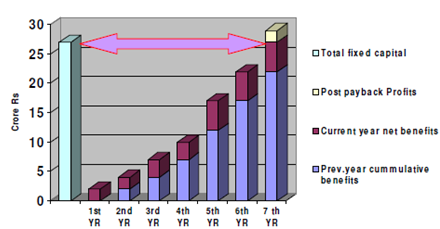## Calculation of Payback Period Assignment Help

Assignment Help: >> Project Investment - Calculation of Payback Period

Calculation of Payback Period:

In order to calculate the net benefits, the interest burden every year is required to be calculated as follows:

(Interest)n      =          (Outstanding Debt)n x Rate of Interest

where  n         =          the year of calculation

Outstanding debt     =          (Previous year outstanding debt) - (current year repayments of loan)

The interest so calculated is deducted from gross benefits to give the "taxable benefits". These are again deducted for tax liabilities at the applicable tax rate to give net benefits:

(Net benefit)  =          [Gross benefit - (Interest)] x (1 - tax rate in %)

The net benefits so arrived at are cumulated and compared till the time they total-up to match the "total fixed cost". The number of such years taken for the cumulative net benefits to exactly match the total fixed cost is the payback period:

Graphically, this process is depicted in below diagram.Figure: Calculating Payback Period

The number 'n' is such that (cumulative net benefits)n    > Z

Then PBP = (n - 1) +[Z - (cumulative Net benefit)n - 1]/ (net benefit)n#### Assured A++ Grade

Get guaranteed satisfaction & time on delivery in every assignment order you paid with us! We ensure premium quality solution document along with free turntin report!

All rights reserved! Copyrights ©2019-2020 ExpertsMind IT Educational Pvt Ltd